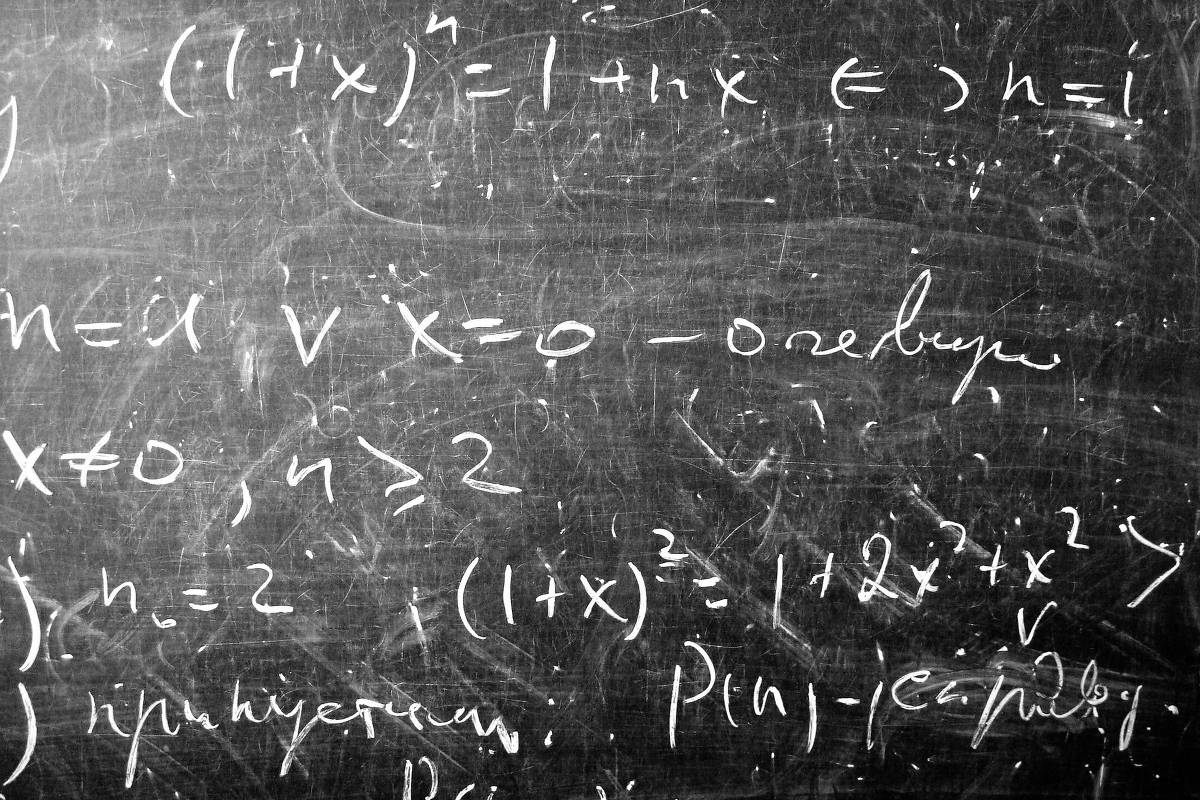Home sci-tech The Strange Case of 6174

# The Strange Case of 6174### Today´s Deals

I found out in college, reading the Mathematical Games column, which the great publicist Martin Gardner (1914 – 2010) published for 25 years in the Scientific American magazine. I spent weeks scribbling billstrying to understand the mystery …

Consider an N number with four digits that are not all the same: for example N = 4347. Arrange the digits in descending (7443) and ascending (3447) order, and call K (N) the difference: K (4347) = 7443-3447 = 3996. This operation was invented in 1949 by the Indian Dattareya Kaprekar (1905 "" 1986), a mathematics teacher in basic education who devoted his life to studying properties of whole numbers. He discovered several startling facts.

To begin with, there is a unique number N that is fixed for the operation, ie, such that K (N) = N. This is 6174, a number to which, frankly, no one had given the slightest ball yet. But there is more. If we repeat the operation, we always end up at the fixed number: for example, K (3996) = 6264, K (6264) = 4176, and K (4176) = 6174. This happens in a maximum of 7 steps, whatever the starting number. Why?

Mathematicians seek to understand mysteries like this by thinking more generally, varying the problem data to make it more comprehensive. In this case, the first thing that comes to mind is to vary the number of digits.

For numbers with 3 digits, the conclusions are similar: there is a single fixed number, 495, and all others (whose digits are not all the same) end up in it. But from there, things get more complicated: for 2 or 5 digits there is no fixed number, and for 6 digits there are two: 549945 and 631764.

Most people's reaction is disappointment. Is the case of the 6174 a mere coincidence and there is no pretty general rule behind it? Before we come to any hasty conclusions, note that Kaprekar's operation does not depend directly on N but on its digits. So if we change numbering base B (we talked about bases last week) the result changes too. And the problem is very interesting.

For 4 digits, there is a fixed number if and only if the base is a multiple of 5. This includes the case B = 10 we started with. For 3 digits, the base must be even, which is also the case with B = 10. For 5 digits, fixed number exists only if B = 2 or B is a multiple of 3. Note that B = 10 is excluded.

And for 2 digits, dear reader, on what bases is there a fixed number? He is unique? Replies are welcome by email: viana.folhasp@gmail.com.

(tagsToTranslate) math (t) accounts (t) sheet

### Recommended Shopping#### Related Articles

This website uses cookies to improve your experience. We'll assume you're ok with this, but you can opt-out if you wish. Accept Read More